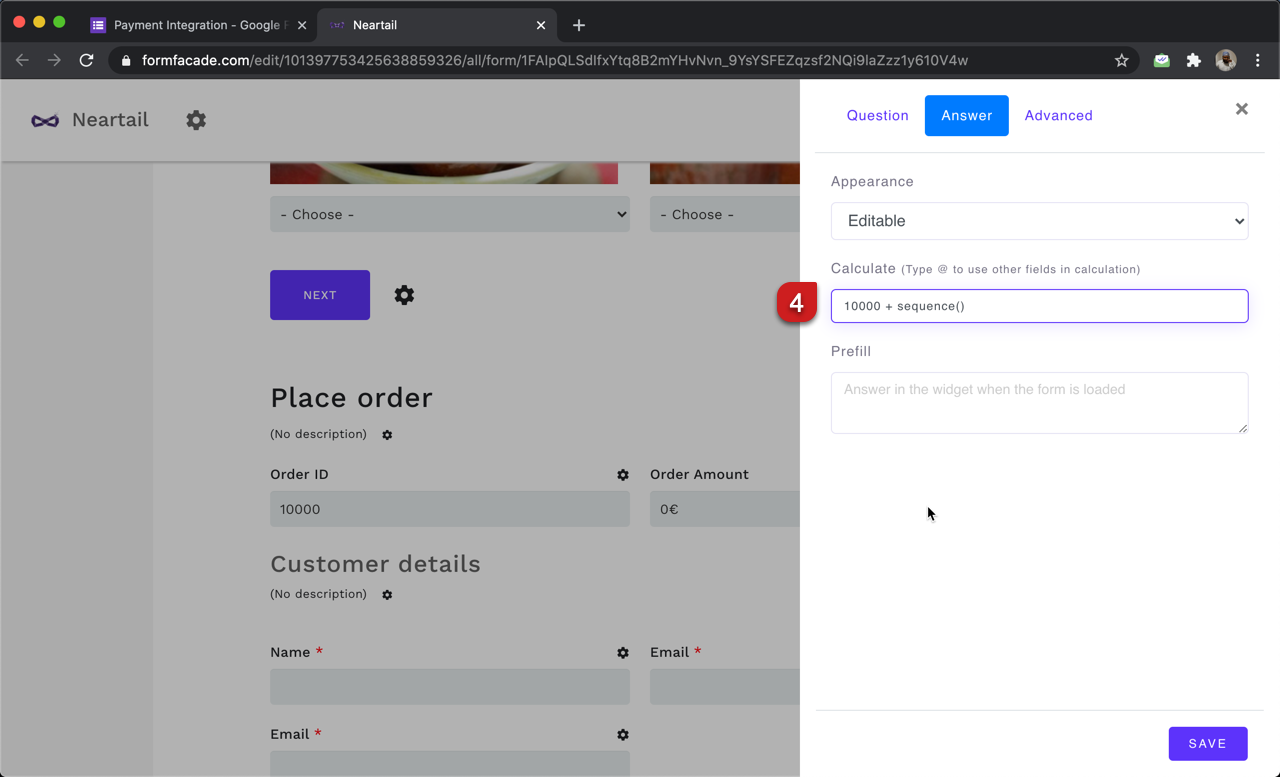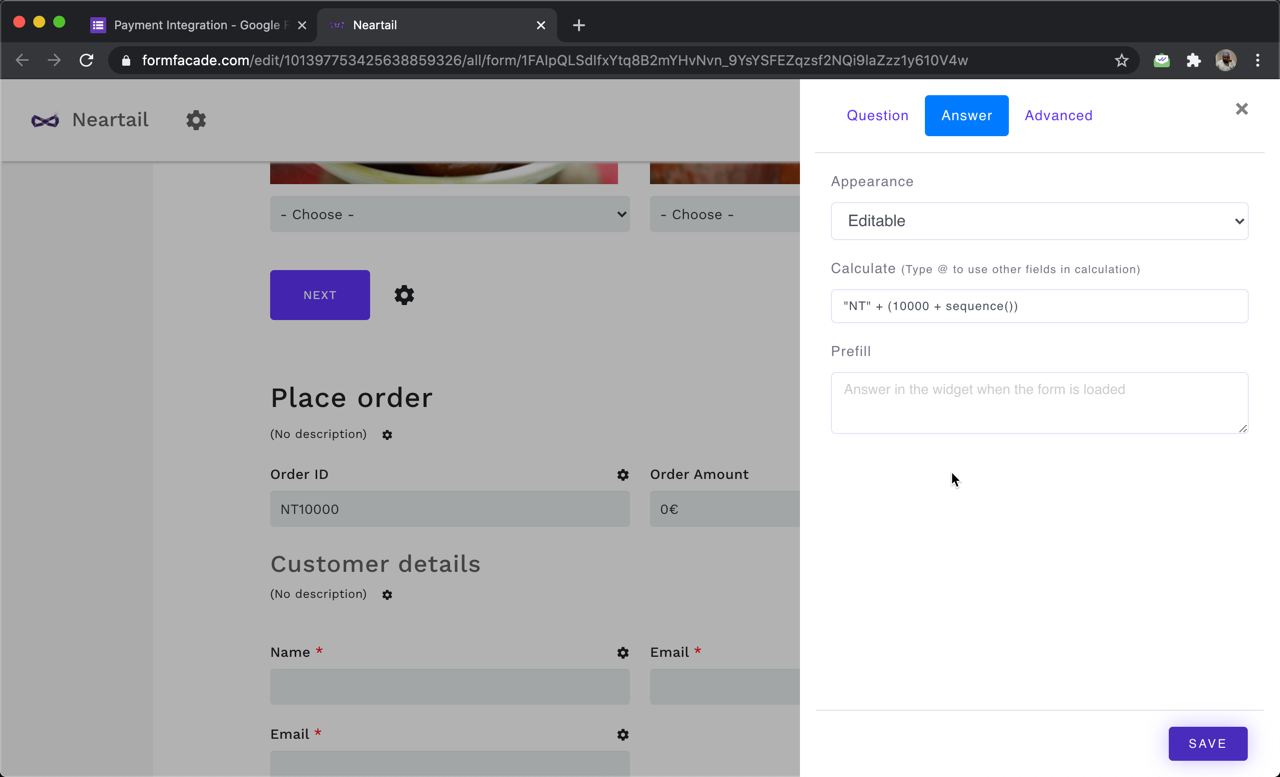### You can use the SEQUENCE() function to generate an order number that is auto incremented by 1 or concatenate it with a text to generate a unique order id.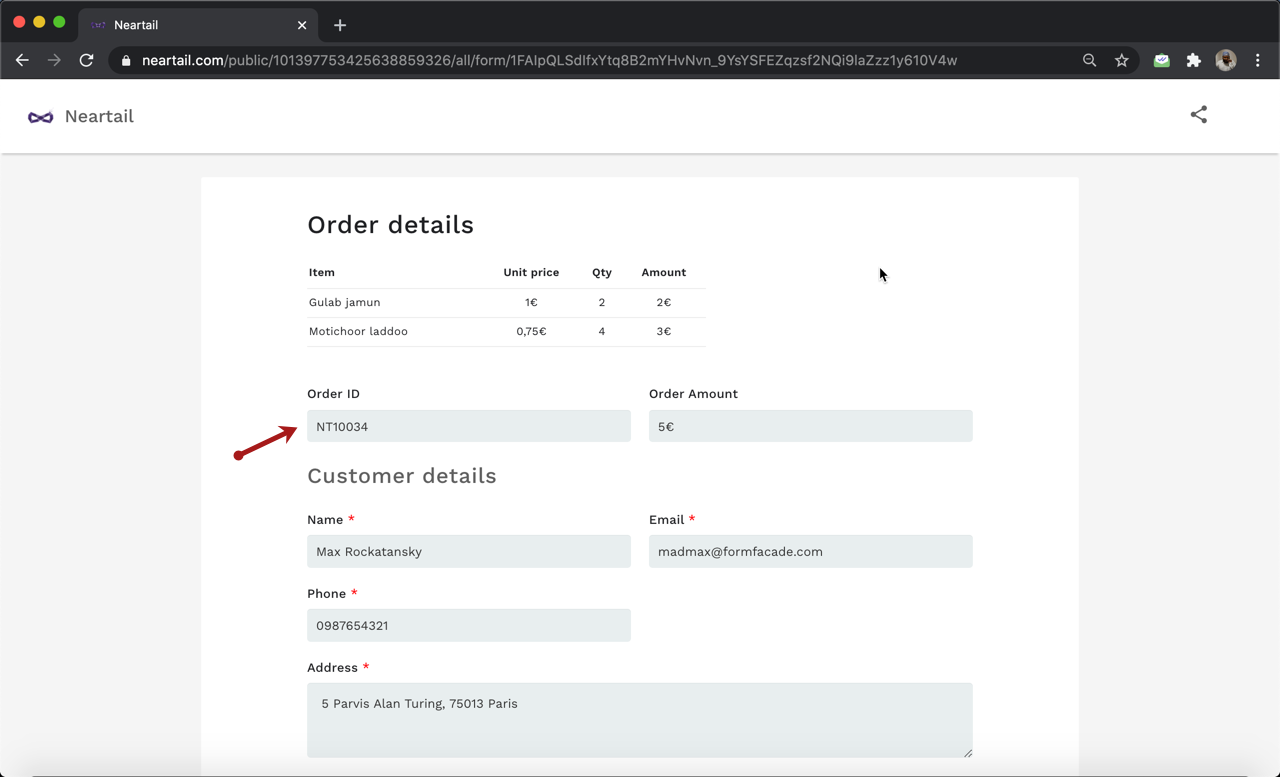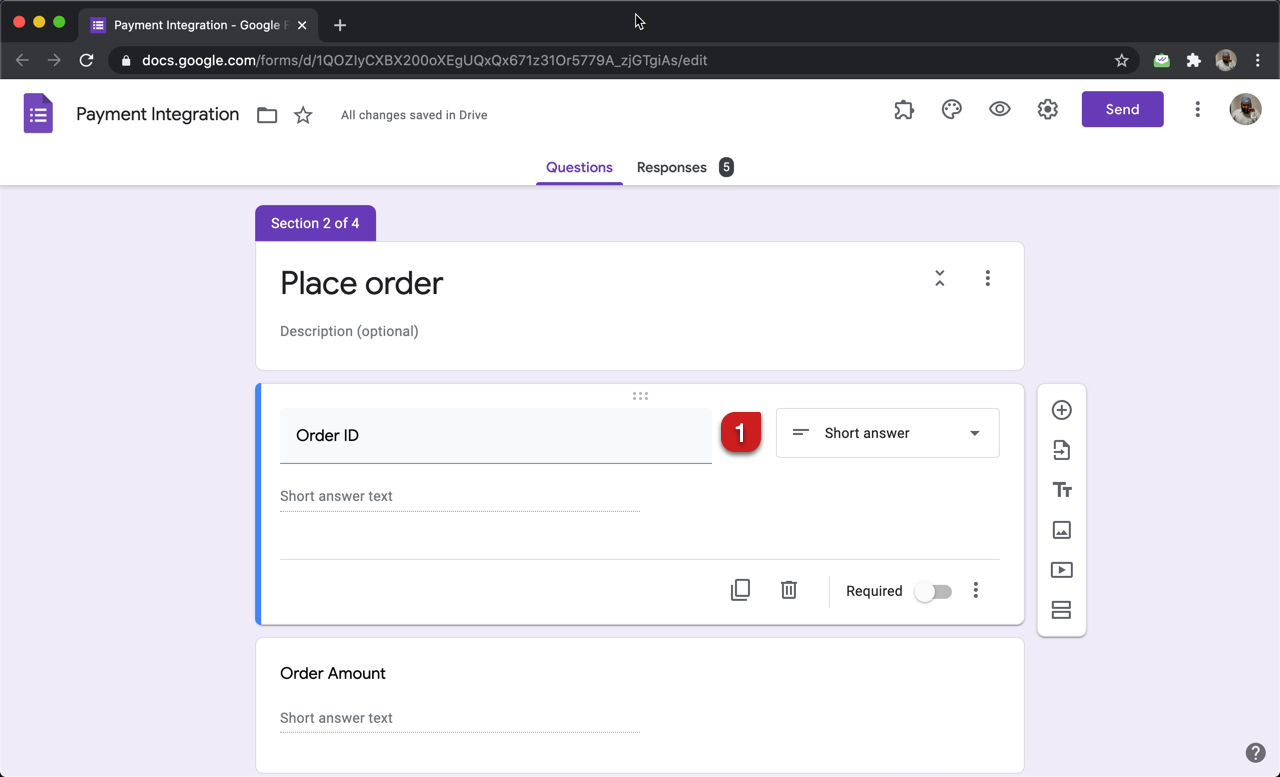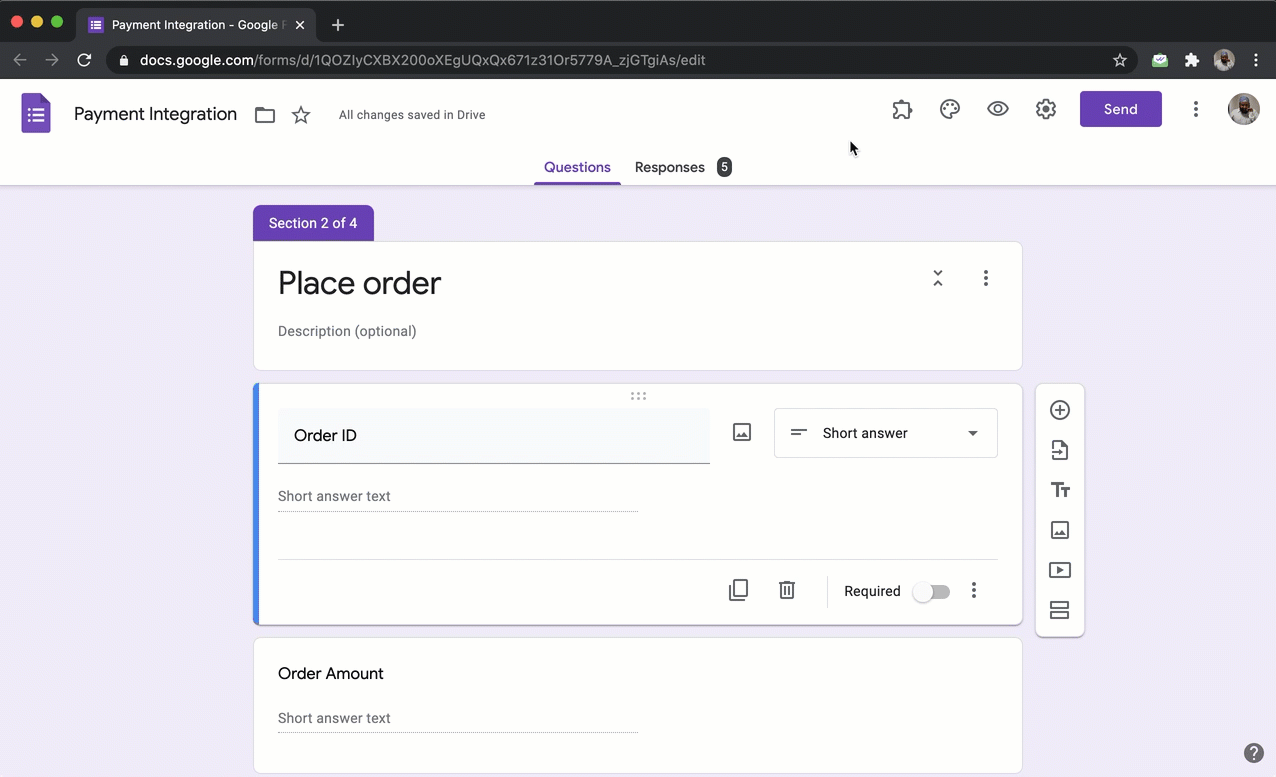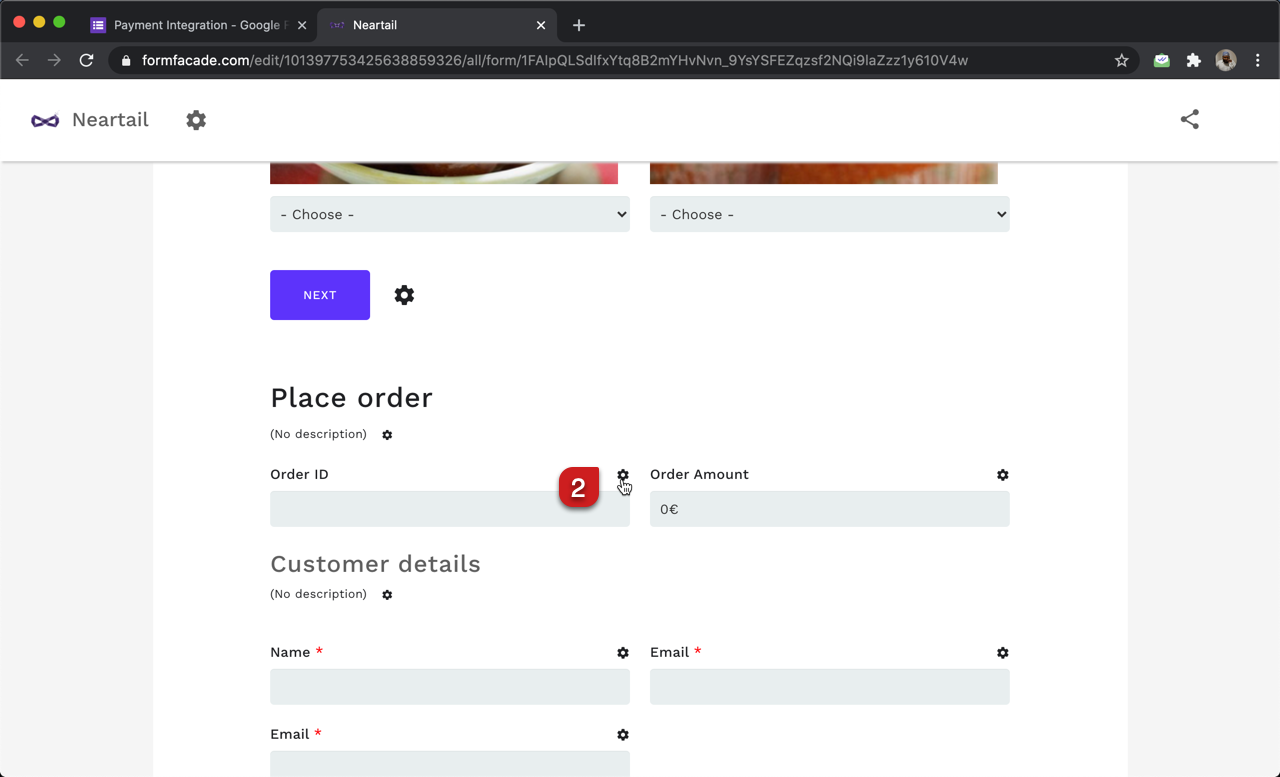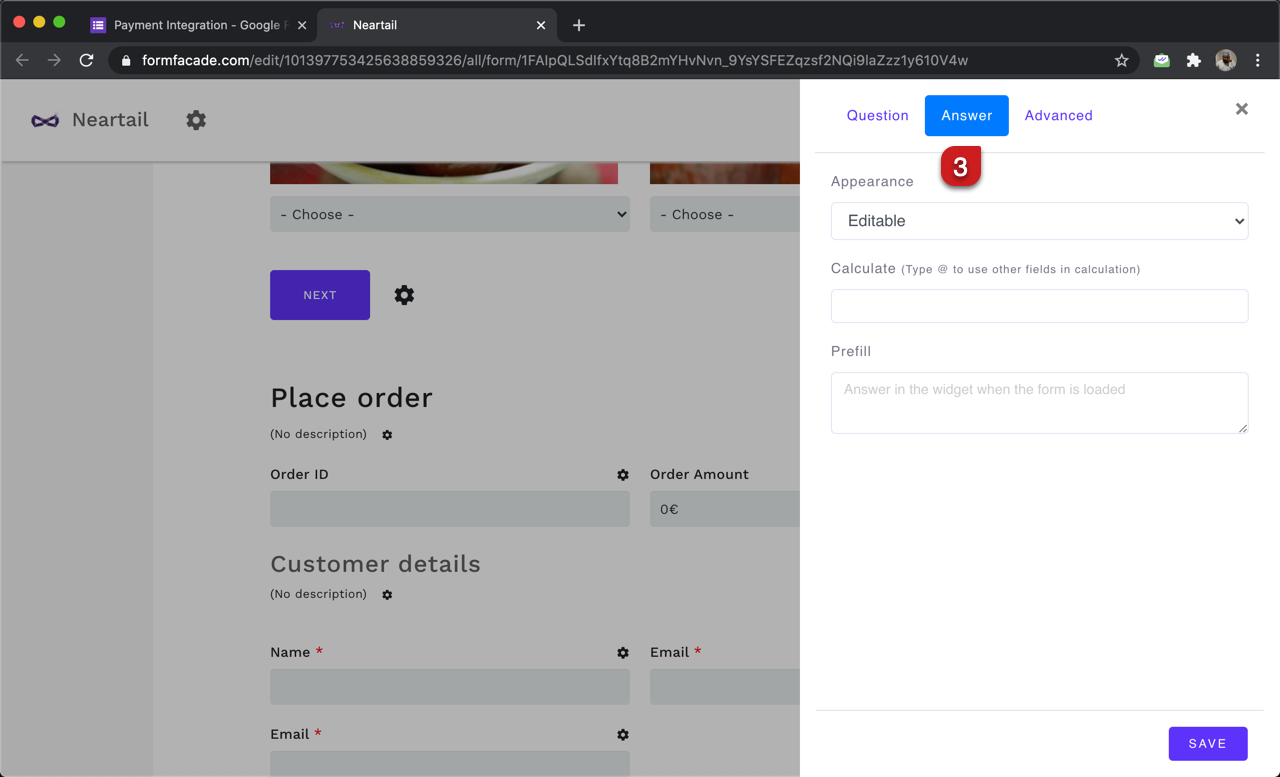#### Use SEQUENCE function to generate order number

SEQUENCE function generates a number that is auto incremented by 1 for each response. If you would like the order number to start from 10001, then you can add the below formula in the calculate option:

10000 + sequence()

Please note that the order number is generated when the user starts filling the form and not when the user submits the form. Hence the order number for the submitted orders may not be sequence. The missing sequence represents the orders that were abandoned by the users.

You can also add a text identifier to generate an order id instead of just a number. For example, you can append "NT" with the order number to generate a unique order id such as NT10001 etc. The formula for generating this order id would be as follows:

"NT" + (10000 + sequence())